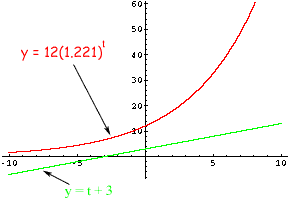Quandaries and Queries JJ Student Secondary Level Questions   A question on my homework assignment about logs says "suppose that x=logA and y=logB & write the expression in terms of x and y" log(A - B) Is this even possible? It seems like a typo.   Another is: "solve for t."   12(1.221)t = t + 3   Is this one possible? If it is, I cant figure it out. Hi JJ, I think you are correct about the first problem. I expect that the expression that was to be rewritten was intended to be log(A/B). Usually, when you have an algebraic expression with the variable in an exponent and you are asked to solve for the variable, the best you can do is approximate a solution. The problem you have, however, has no solution. I convinced myself of this by plotting y = 12(1.221)t and y = t + 3 on the same graph and observing that they don't intersect.Penny Go to Math Central